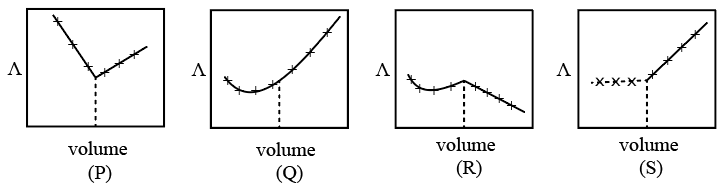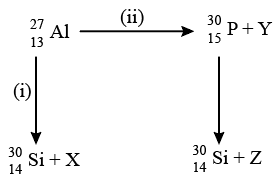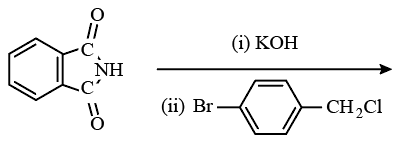Instructions

For the following questions answer them individually

Question 1

# Geometrical shapes of the complexes formed by the reaction of $$Ni^{2+}$$ with $$Cl^-, CN^-$$ ans $$H_2O$$, respectively, areQuestion 2

# $$AgNO_3$$ (aq.) was added to an aqueous KCl solution gradually and the conductivity of the solution was measured. The plot of conductance $$(\bigwedge)$$ versus the volume of $$AgNO_3$$ is-Question 3

# Bombardment of aluminum by $$\alpha$$- particle leads to its artificial disintegration in two ways, (i) and (ii) as shown. Products X, Y and Z respectively are-Question 4

# Extra pure $$N_2$$ can be obtained by heating-Question 5

# Among the following compounds, the most acidic is -Question 6

# The major product of the following reaction is -Question 7

# Dissolving 120 g of urea (mol. wt. 60) in 1000 g of water gave a solution of density 1.15 g/mL. The molarity of the solution is -Question 8

# Extraction of metal from the ore cassiterite involves -Question 9

# Amongst the given options, the compound(s) in which all the atoms are in one plane in all the possible conformations (if any), is (are)Question 10

# The correct statement(s) pertaining to the adsorption of a gas on a solid surface is (are)OR